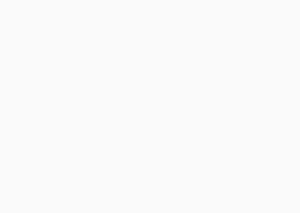#IBPS Clerk Prelims: Quants Day 10

Hello Aspirants. Welcome to Online Quant Section in AffairsCloud.com. We are starting IBPS Clerk course 2015 and we are creating sample questions in Quantitative Aptitude section, type of which will be asked in IBPS Clerk Prelims Exam.

Stratus – IBPS Clerk Course 2015[flipclock]

Directions (1-5): In each of these questions, two equations are given. You have to solve these equations and find out the values of x and y and give answer

1. 3x2 – 14x + 8 = 0, 3y2 + 2y – 8 = 0
A) x > y
B) x < y
C) x ≥ y
D) x ≤ y
E) x = y or relationship cannot be determined
E) x = y or relationship cannot be determined
Explanation:

3x2 – 14x + 8 = 0
3x2 – 12x – 2x + 8 = 0
3x(x-4) – 2(x-4) = 0
x = 2/3, 4
3y2 + 2y – 8 = 0
3y2 + 6y – 4y – 8 = 0
3y(y+2) – 4(y-2) = 0
y = 4/3, 2

2. 8x2 + 6x = 5, 12y2 – 22y + 8 = 0
A) x > y
B) x < y
C) x ≥ y
D) x ≤ y
E) x = y or relationship cannot be determined
D) x ≤ y
Explanation:

8x2 + 6x – 5 = 0
8x2 + 10x – 4x – 5 = 0
2x(4x+5) – (4x+5) = 0
x = 1/2 , -5/4
12y2 – 22y + 8 = 0
12y2 – 16y – 6y +8 = 0
4y(3y-4) – 2(3y-4) = 0
y = 1/2, 4/3

3. x = √172, y2 – 29y + 210 = 0
A) x > y
B) x < y
C) x ≥ y
D) x ≤ y
E) x = y or relationship cannot be determined
B) x < y
Explanation:

x = √172 = 13.1
y2 – 29y + 210 = 0
(y-15)(y-14) = 0
y = 14,15

4. 7x + 16y = 75, 6x – 8y = -9
A) x > y
B) x < y
C) x ≥ y
D) x ≤ y
E) x = y or relationship cannot be determined
B) x < y
Explanation:

Solve the equations, x = 3, y = 27/8

5. x2 + 11x + 30 = 0, y2 + 12y + 36 = 0
A) x > y
B) x < y
C) x ≥ y
D) x ≤ y
E) x = y or relationship cannot be determined
C) x ≥ y
Explanation:

x2 + 11x + 30 = 0
(x+6)(x+5) = 0
x = -5, -6
y2 + 12y + 36 = 0
(y+6)2 = 0
y = -6

Directions (Q. 6-10): Study the following graph and answer the following questions.1. In which year was the sale of ‘Pep-up’ the maximum?
A) 2011
B) 2012
C) 2013
D) 2010
E) None of these
D) 2010
Explanation:

It can be seen in graph that in 2010 it is max.

2. In the case of which soft drink was the average annual sale maximum in the given period?
A) Pep-up only
B) Cool-sip only
C) Dew-drop only
D) Cool-sip and Dew-drop
E) None of these
A) Pep-up only
Explanation:

Add the sales of all dinks individually. The drink which has max in total is the answer.

3. In the case of Cool-sip drink, what was the percent increase in sale in 2013 over its sale in 2012?
A) Less than 20
B) 20-25
C) 25
D) 31-35
E) None of these
C) 25
Explanation:

25-20/20 * 100 = 25%

4. What was the approximate per cent increase in sale of all drinks in 2013 over its sale in 2010?
A) 51%
B) 45%
C) 22%
D) 32%
E) None of these
D) 32%
Explanation:

Sales in 2013 = 30+25+20 = 75
Sales in 2010 = 15+7+35= 57
So required % = 75-57/57 * 100 = 32%

5. What was the approximate per cent drop in sale of Pep-up in 2011 over its sale in 2010?
A) 5
B) 12
C) 14
D) 20
E) None of these
C) 14
Explanation:

35-30/35 * 100

AffairsCloud Recommends Oliveboard Mock Test

AffairsCloud Ebook - Support Us to Grow